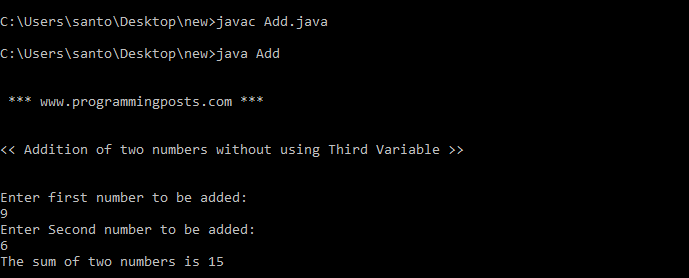# Java Program to add two numbers without using third variable

In this program user is asked to enter two integers and the sum operation is performed.

### Source code:

```import java.util.*;
class Add
{
public static void main(String args[])
{
int num1;
int num2;
System.out.println("\n \n *** www.programmingposts.com *** \n \n");
System.out.println("<< Addition of two numbers without using Third Variable >> \n\n ");
Scanner scanner = new Scanner(System.in);
System.out.println("Enter first number to be added:");
num1 = scanner.nextInt();
System.out.println("Enter Second number to be added:");
num2 = scanner.nextInt();
num1 = num1 + num2;
System.out.println("The sum of two numbers is " +num1);
}
}```

### Output:

C:\Users\Desktop\new>javac  Add.javaC:\Users\Desktop\new>java Add

*** www.programmingposts.com ***

<< Addition of two numbers without using Third Variable >>

Enter first number to be added:
9
Enter Second number to be added:
6
The sum of two numbers is 15

### Screenshot of output:Screenshot of output